CAT  >  Questions with Answers: Probability

# Questions with Answers: Probability Notes - CAT

## Document Description: Questions with Answers: Probability for CAT 2022 is part of CAT preparation. The notes and questions for Questions with Answers: Probability have been prepared according to the CAT exam syllabus. Information about Questions with Answers: Probability covers topics like and Questions with Answers: Probability Example, for CAT 2022 Exam. Find important definitions, questions, notes, meanings, examples, exercises and tests below for Questions with Answers: Probability.

Introduction of Questions with Answers: Probability in English is available as part of our CAT preparation & Questions with Answers: Probability in Hindi for CAT courses. Download more important topics, notes, lectures and mock test series for CAT Exam by signing up for free. CAT: Questions with Answers: Probability Notes - CAT
 1 Crore+ students have signed up on EduRev. Have you?

# Q.1. If 4 whole numbers taken at random are multiplied together show that the chance that the last digit in the product is 1,3,7, or 9 is 16/625.

If the last digit is 1,3,7,9 in the product then we can only select numbers
whose last digit is 1,3,7 or 9 from 0,1,2,.....9
Probability that a selected number  ends with 1,3,7 or 9  = 4/10 = 2/5
Probability of selecting four such numbers = (2/5)= 16/625

Q.2. It is given that the events A and B are such that P(A) = 1/4, P(A|B) = 1/2 and P(B|A) = 2/3. Then P(B|A) = 2/3. Then P(B) is
(a) 1/2
(b) 1/6
(c) 1/3
(d) 2/3

Given that P(A) = 1/4,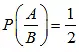and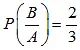we know,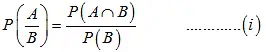andNow, substitute the values in the formula, we get

(2/3) = P(B∩A)/ (1/4)

P(B∩A) = (2/3). (1/4)

P(B∩A) = 1/6

Substitute the values in (1), we get

P(A/B) = P(A∩B)/ P(B)

(1/2) = (1/6)/ P(B)

P(B) = (1/6). (2/1)

P(B) = 1/3

Q.3. A die is thrown. Let A be the event that the number obtained is greater than 3. Let B be the event that the number obtained is less than 5. Then P (AUB) is
(a) 2/5
(b) 3/5
(c) 0
(d) 1

Since,
A =  number > 3
A {4,5,6}
and B = number < 5
B = {1, 2, 3, 4}
∴ A∩B = {4}

∴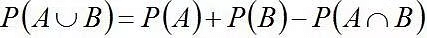⇒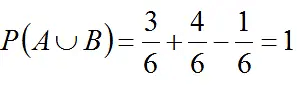Q.4. A pair of fair dice is thrown independently three times. The probability of getting a total of exactly 9 twice is
(a) 1/729
(b) 8/9
(c) 8/729
(d) 8/243

When two dice are thrown, the total number of sample space is 36
Probability of getting a total of 9 in a single throw
4/36 = 1/9
Hence, the probability of getting a score of exactly 9 twice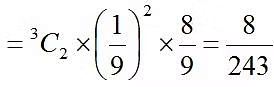Q.5. Two aeroplanes I and II bomb a target in succession. The probabilities of I and II scoring a hit correctly are 0.3 and 0.2, respectively. The second plane will bomb only if the first misses the target. The probability that the target is hit by the second plane, is
(a) 0.06
(b) 0.14
(c) 0.32
(d) 0.7

Let the events,
A = 1st aeroplane hit the target
B =2nd aeroplane hit the target
And their corresponding probabilities are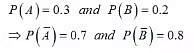Required Probability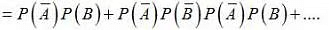= (0.7)(0.2) + (0.7)(0.8)(0.7)(0.2)+ (0.7)(0.8)(0.7)(0.8)(0.7)(0.2) + ....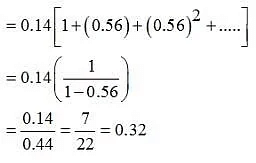The document Questions with Answers: Probability Notes - CAT is a part of CAT category.
All you need of CAT at this link: CAT
 Use Code STAYHOME200 and get INR 200 additional OFF

### How to Prepare for CAT

Read our guide to prepare for CAT which is created by Toppers & the best Teachers

Track your progress, build streaks, highlight & save important lessons and more!

,

,

,

,

,

,

,

,

,

,

,

,

,

,

,

,

,

,

,

,

,

;